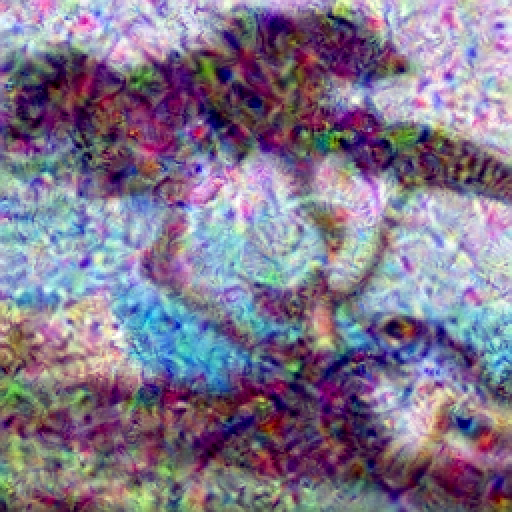#Function Repository Resource:

# DeepDreamBeta

Render an image using the DeepDream-β algorithm

Contributed by: Aster Ctor  |  Aster Ctor (MoeNet)
 ResourceFunction["DeepDreamBeta"][net,image] renders the image using the deepdream-β algorithm. ResourceFunction["DeepDreamBeta"][net,image,step] renders the image with an iteration depth of step.

## Details and Options

ResourceFunction["DeepDreamBeta"] has the following options:
 "Eyes" 2 network activation depth "Activation" Identity pre-activation function for image "StepSize" 1 step size of each pattern overlay iteration Resampling "Cubic" works the same as ImageResize TargetDevice "CPU" works the same as NetChain WorkingPrecision "Real32" works the same as NetChain

## Examples

### Basic Examples (3)

Take a neural network as the dreamer:

 In:=Out=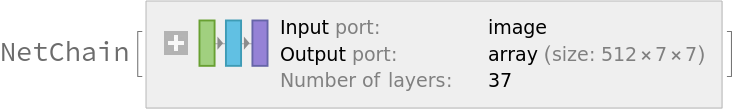In:=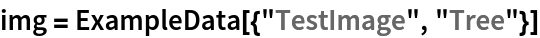Out=Now process it with DeepDreamBeta:

 In:=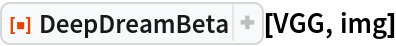Out=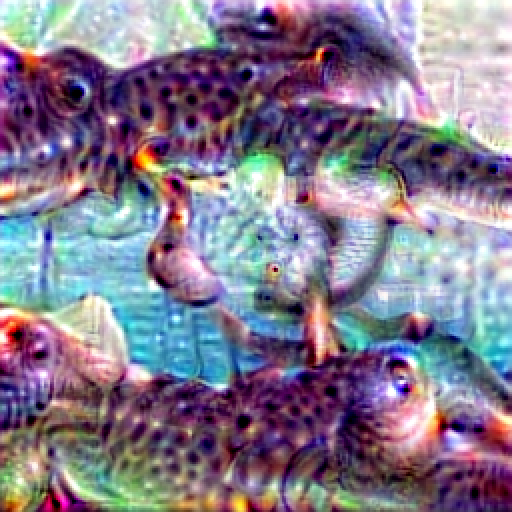### Scope (3)

Take a neural network as the dreamer:

 In:=Out=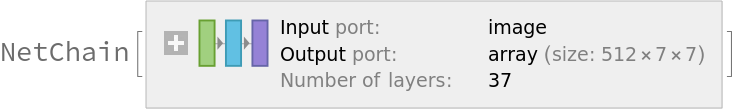In:=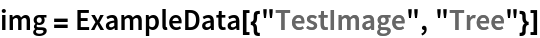Out=Use GPU acceleration:

 In:=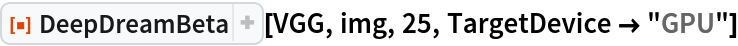Out=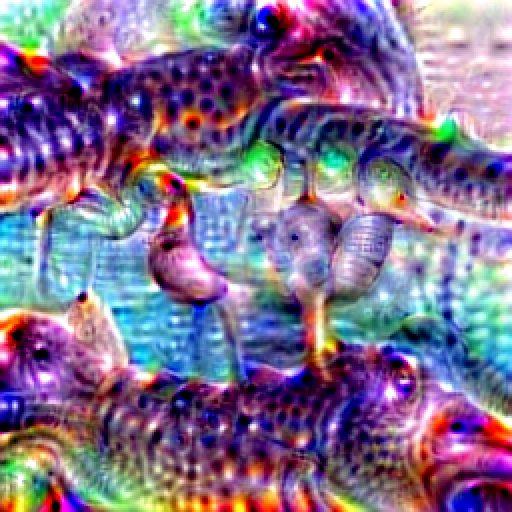### Options (10)

#### Activation (3)

Take a neural network as the dreamer:

 In:=Out=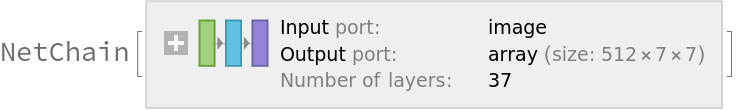In:=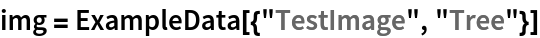Out=Change the pre-activation function:

 In:=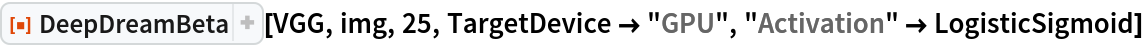Out=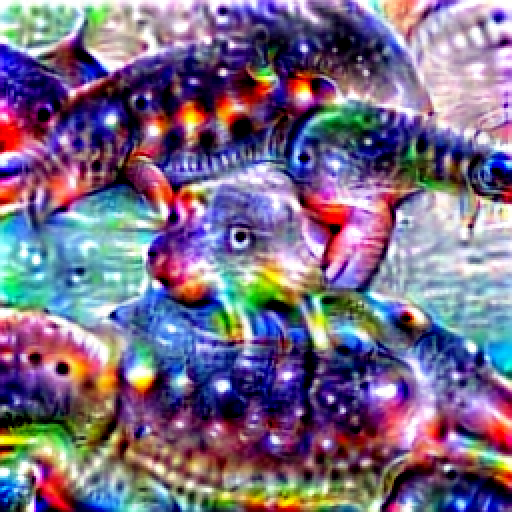#### Eyes (4)

Take a neural network as the dreamer:

 In:=Out=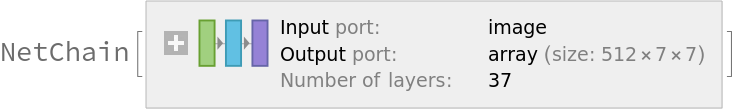In:=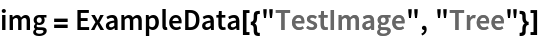Out="Eyes" controls the size of the receptive field:

 In:=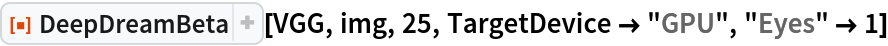Out=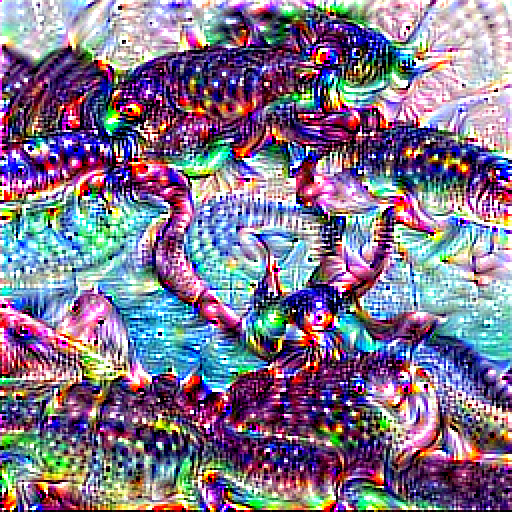More eyes gives a greater departure from the original picture:

 In:=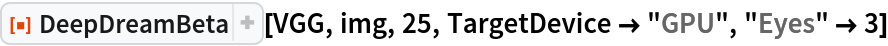Out=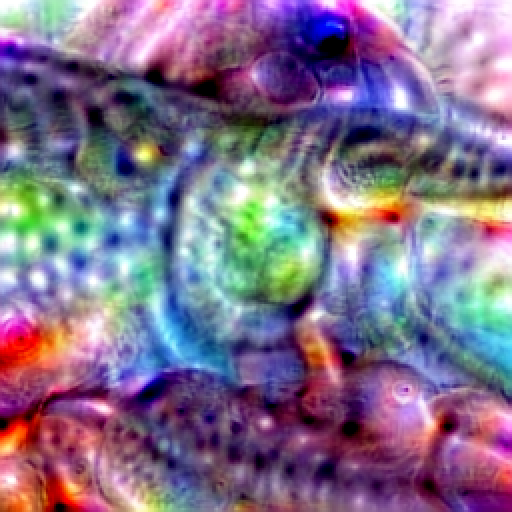#### StepSize (3)

Take a neural network as the dreamer:

 In:=Out=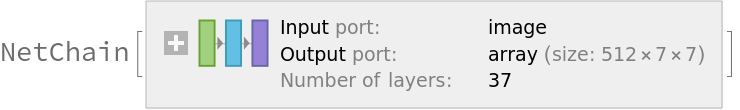In:=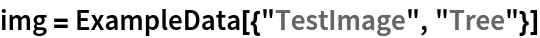Out=The smaller the step, the smoother the final result will be:

 In:=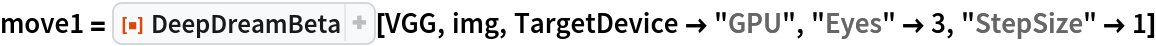Out=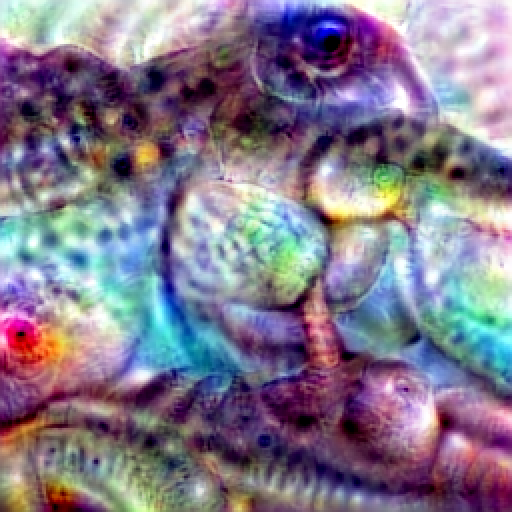In:=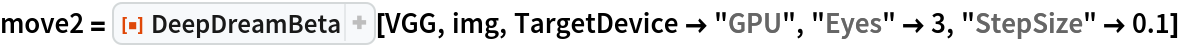Out=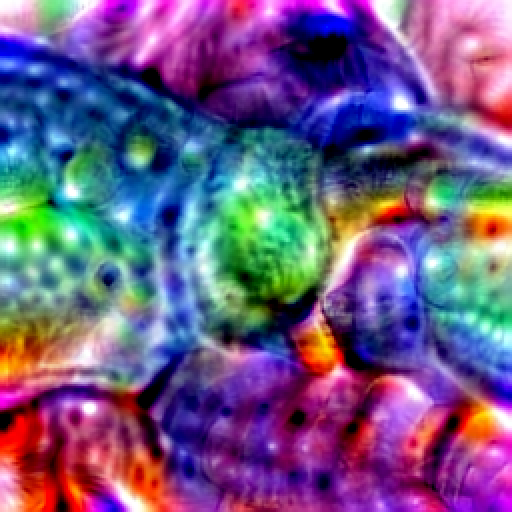### Properties and Relations (2)

Use non-residual networks for better results.

The residual activation network is difficult to activate abstract features:

 In:=Out=Generate messy textures:

 In:=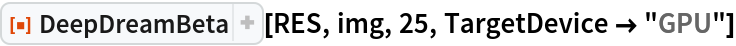Out=